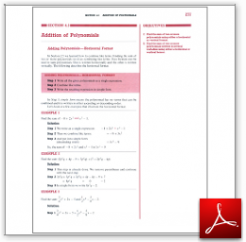Algebra Math Book - Introductory Algebra Thank you for your continual support.

 The power of mathematics rests in the logic of thinking.ID: Sec4-3
Description: Adding in Horizontal Format-Vertical Format-
Price: 1.99

# Algebra Math book - Introductory Algebra - Chapter 4 - Section 3 - Addition of Polynomials

## Section 4.3 - Objectives

7.  Find the sum of two or more polynomials using either a horizontal or vertical format.

8.  Find the sum of two or more polynomials written in several variables using either a horizontal or vertial format.

Navigate to
Previous Section or Next Section
or Chapter 4 Details or Other Chapters

This section of my algebra math book, Introductory Algebra, also includes in the download:

• Cover Sheet